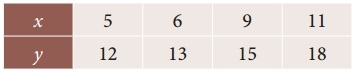Tamilnadu State Board New Syllabus Samacheer Kalvi 12th Business Maths Guide Pdf Chapter 5 Numerical Methods Ex 5.3 Text Book Back Questions and Answers, Notes.

## Tamilnadu Samacheer Kalvi 12th Business Maths Solutions Chapter 5 Numerical Methods Ex 5.3

Choose the most suitable answer from the given four alternatives:

Question 1.
Δ²y0 =
(a) y2 – 2y1 + y0
(b) y2 + 2y1 – y0
(c) y2 + 2y1 + y0
(d) y2 + y1 + 2y0
Solution:
(a) y2 – 2y1 + y0
Hint:
Δ²y0 = (E – 1)²y0
= (E² – 2E + 1) y0
= E²y0 – 2Ey0 + y0
= y2 – 2y1 + y0

Question 2.
Δf(x) =
(a) f (x + h)
(b) f (x) – f (x + h)
(c) f (x + h) – f(x)
(d) f(x) – f (x – h)
Solution:
(c) f (x + h) – f(x)
Hint:
Δf(x) = f (x + h) – f(x)Question 3.
E≡
(a) 1 + Δ
(b) 1 – Δ
(c) 1 + ∇
(d) 1 – ∇
Solution:
(a) 1 + Δ

Question 4.
If h = 1, then Δ(x²) =
(a) 2x
(b) 2x – 1
(c) 2x + 1
(d) 1
Solution:
(c) 2x + 1
Hint:
Δ(x²) = (x + h)² – (x)²
= x² + 2xh + h² – x²
Δ(x²) = 2xh + h²
If h = 1 Δ (x²) = 2x + 1Question 5.
If c is a constant then Δc =
(a) c
(b) Δ
(c) Δ²
(d) 0
Solution:
(d) 0

Question 6.
If m and n are positive integers then Δm Δn f(x)=
(a) Δm+n f(x)
(b) Δm f(x)
(c) Δn f(x)
(d) Δm-n f(x)
Solution:
(a) Δm+n f(x)Question 7.
If ‘n’ is a positive integer Δn-n f(x)]
(a) f(2x)
(b) f(x + h)
(c) f(x)
(d) Δf(x)
Solution:
(c) f(x)
Hint:
Δn-n f(x)] = Δn-n f(x) = Δ0 f(x)
= f(x)

Question 8.
E f(x) =
(a) f(x – h)
(b) f(x)
(c) f(x + h)
(d) f(x + 2h)
Solution:
(c) f (x + h)Question 9.
∇≡
(a) 1 + E
(b) 1 – E
(c) 1 – E-1
(d) 1 + E-1
Solution:
(c) 1 – E-1

Question 10.
∇ f(a) =
(a) f(a) + f(a – h)
(b) f(a) – f(a + h)
(c) f(a) – f(a – h)
(d) f(a)
Solution:
(c) f(a) – f(a- h)Question 11.
For the given points (x0, y0) and (x1, y1) the Lagrange’s formula is
(a) y(x) = $$\frac { x-x_1 }{x_0-x_1}$$ y0 + $$\frac { x-x_0 }{x_1-x_0}$$ y1
(b) y(x) = $$\frac { x_1-x}{x_0-x_1}$$ y0 + $$\frac { x-x_0 }{x_1-x_0}$$ y1
(c) y(x) = $$\frac { x-x_1 }{x_0-x_1}$$ y0 + $$\frac { x-x_0 }{x_1-x_0}$$ y0
(d) y(x) = $$\frac { x_1-x }{x_0-x_1}$$ y0 + $$\frac { x-x_0 }{x_1-x_0}$$ y0
Solution:
(a) y(x) = $$\frac { x-x_1 }{x_0-x_1}$$ y0 + $$\frac { x-x_0 }{x_1-x_0}$$ y1

Question 12.
Lagrange’s interpolation formula can be used for
(a) equal intervals only
(b) unequal intervals only
(c) both equal and unequal intervals
(d) none of these.
Solution:
(c) both equal and unequal intervals.Question 13.
If f(x) = x² + 2x + 2 and the interval of differencing is unity then Δf(x)
(a) 2x – 3
(b) 2x + 3
(c) x + 3
(d) x – 3
Solution:
(b) 2x + 3
Hint:
Given:
f(x) = x² + 2x + 2
Δf(x) = f (x + h) – f (x)
since h = 1
Δf(x) = f (x – 1) – f (x)
= [(x + 1)² + 2(x + 1) + 2] – [x² + 2x + 2]
= [x² + 2x + 1 + 2x + 2 + 2] – [x² + 2x + 2]
= x² + 4x + 5 – x² – 2x – 2
= 2x + 3Question 14.
For the given data find the value of Δ³y0 is(a) 1
(b) 0
(c) 2
(d) -1
Solution:
(b) 0
Hint:
From this data
y0 = 12; y1 = 13; y2 = 15; y3 = 18
Δ³y0 = (E – 1)³ y0
= (E³ – 3E² + 3E – 1) y0
= E³y0 – 3E²y0 + 3Ey0 – y0
= y3 – 3y2 + 3y1 – y0
= 18 – 3 (15) + 3 (13) – 12
= 18 – 45 + 39 – 12
= 57 – 57 = 0